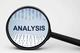# EBITDA to Interest Coverage Ratio

By Research DeskEBITDA to Interest Coverage Ratio is a measure used to understand the company’s ability to service its liabilities (debts and leases) from operating profits. It takes into consideration the EBITDA or operating profits of the firm to the interest expenditure.

The formula used to determine the EBITDA to Interest Coverage Ratio is:

EBITDA to Interest Coverage Ratio = EBITDA / Interest Expenses

Where EBITDA is Earnings Before Interest, Tax, Depreciation, Amortization and is calculated using Net Income + Tax + Interest + Depreciation + Amortization.

Since EBITDA gives us a better outlook of the cash earnings of the company (since depreciation and amortisation is a non-cash item) and all the interest payments have to be done in cash, this ratio helps us to determine a more useful source of a company’s ability to service debt obligations.

For better understanding let us take an example through the financials of a company, say ABC Ltd.

 ABC Ltd. Net Profit: Rs. 844 crore Tax: Rs. 115 crore Interest Expense: Rs. 143 crore Depreciation: Rs. 121 crore Amortization: Rs. 3 crore Thus, First calculate EBITDA EBITDA = Net Income + Tax + Interest Expense + Depreciation + Amortization               = 844 + 115 + 143 + 121 + 3               = 1,226 crore Thus EBITDA to Interest Coverage Ratio is 3,226 / 143 = 8.6 times

Let’s say that the industry average of the EBITDA to Interest Coverage Ratio is 6, then having a high EBITDA to Interest Coverage Ratio signifies that the company is having better cash earnings (like for ABC Ltd. above) than peers to service debt and is not overburdened with debt obligations.